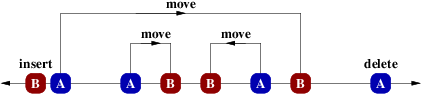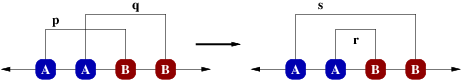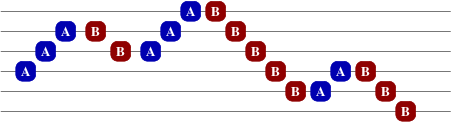(Analysis by Brian Dean)

Letting $K = 10$ be the maximum amount of dirt in any cell, we can ultimately solve this problem using dynamic programming in $O(NK)$ time, which runs in less than 0.1 seconds on every test case. However, it takes a number of structural insights to get to this point. There are several ways to attack this problem, many based on more advanced network flow techniques like min-cost flows, which are perhaps slightly beyond the typical scope of USACO contests. For simplicity, we focus here on a solution that only uses dynamic programming, and no advanced flow techniques. We may soon add extra detail from other solutions that use flow-based techniques, for completeness.

For starters, it may help to review the $O((NK)^2)$ solution of the earlier version of this problem, which appeared on the silver 2012 March USACO contest. As in that problem, we first unpack each array into a list of $O(NK)$ dirt locations. For example, a landscape with heights 3,1,4,1 turns into the sequence 0,0,0,1,2,2,2,2,3 (e.g., there are 4 units of dirt at position 2). The previous solution involved using an "edit distance" style DP algorithm to transform the initial sequence of dirt locations into the target sequence in quadratic time.

It will be convenient to visualize this as matching a set of points on a number line, where the As represent locations of the source dirt and Bs are the target dirt locations. If we match an A with a B (at distance $d$), this costs $dZ$ and corresponds to moving a piece of dirt. Unmatched As correspond to deletions (cost $Y$) and unmatched Bs correspond to insertions (cost $X$).It will be helpful to assume that we match all of the smaller set of points; for example, if there are fewer As, we'd like to assume that we want to match all the As, leaving only Bs unmatched. To do this, we set the cost of matching a distance-$d$ pair to be $\min(dZ, X+Y)$, reflecting now the possibility that the matched pair might not be an actual move, but may instead be a "teleport" caused by a delete plus an insert. We can now assume that all elements of the smaller set must be matched.

A key structural property is that there always exists an optimal matching with a "nesting" structure, where pairings can nest but not "cross". This follows from the fact that any set of crossing edges can be "uncrossed", for example as shown below, without harming (increasing) the total cost of the matching. By doing this repeatedly, we can turn any optimal matching into an optimal matching with no crossings.Recall that the cost of a distance-$d$ edge in the matching is $\min(dZ, X+Y)$. If the cost is $X+Y$, we'll call the edge "long"; otherwise it is "short". We can prove that cost doesn't increase by the uncrossing operation by breaking it into three cases: (i) both p and q are short, (ii) one of p and q is long, (iii) both p and q are long. In all cases, you can easily show that the total cost does not increase. For example, in case (iii), edge s must also be long, so the contribution before uncrossing is $2(X+Y)$ and the contribution after is $X+Y+\min(dr, X+Y) \leq 2(X+Y)$.

Non-crossing implies also that underneath each edge must be the same number of As and Bs. Assume for a moment (without loss of generality) that there are more Bs than As, so all As must be matched. In this case, if there are more As than Bs underneath edge p as shown below, then one of the As would need to be matched in a way that crosses p.Similarly, if there are more Bs than As underneath edge q, then one of them must remain unmatched (since otherwise there would be a crossing, just as before), and then we could decrease the length of edge q by linking with the unmatched B inside the range, giving an even more optimal solution (or at least, not a worse solution).

Let us now assign heights to our points, with successive As moving upward and Bs moving downward:The fact that the points underneath each edge must be balanced therefore implies that we can decompose our problem and consider each height level independently. This simplifies things quite a bit, since within any given height level we have alternating As and Bs. Either we have the same number of As and Bs and they are all matched, or we have one excess element that is unmatched while the rest are matched. In this second case, one can show that an optimal solution will not involve an edge across the unmatched element, so it further decomposes into a prefix of balanced As and Bs (all matched to each-other), followed by the unmatched element, followed by a suffix of balanced As and Bs, all matched:We therefore have reduced our problem to: given an alternating set of points, find the optimal way to match every balanced prefix (and by symmetry, suffix). We show how to do this in linear time with dynamic programming, after which another linear-time scan can be used to find the right way to combine the two solutions as in the "one element unmatched" case above.

Our dynamic programming formulation looks like the following. For each balanced prefix (say, up to position $j$), we compute the optimal way to match all its elements, and also the optimal way to match its elements such that $j$ is matched with a long edge. For matching element $j$ with a long edge, candidates to which we can match are those elements in regions $R$ and $S$ in the figure below:We can afford to check all the candidates in region $S$ explicitly, since for all values of $j$ this will involve monotonically scanning the entire line just once. Each candidate $i$ in $S$ can be checked in $O(1)$ time, since we have already computed the best way to match everyone up to $i-1$, and since the elements underneath the long $i$-$j$ edge can be assumed to be simply paired up consecutively (so this cost can be computed in constant time using the difference of two prefix sums). This follows from the observation that an optimal solution will never involve a long edge nested within another long edge, so underneath any long edge we have only short edges; furthermore, nested short edges can always be uncrossed with no change in objective value, so we can assume the short edges underneath a long edge are just consecutively paired, with no nesting at all. To evaluate candidates in the $R$ region, we note that the best such candidate is the same as the best long-edge match from element $j-2$, the only difference being that its cost has increased by the additional cost of pairing elements $j-2$ and $j-1$.

For matching element $j$ with a short edge, we can assume (by the reasoning above, since short edges don't nest), that $j$ would be paired with $j-1$, so this is easy to evaluate in $O(1)$ time. This completes the description of the DP algorithm.

My code is below. The input part in the main function arranges elements by level, then the solve() function runs independently within each level, calling the DP code once or twice (twice if we need to combine a prefix and a suffix solution, having to deal with an odd number of elements on some level). The DP code is a bit cryptic, but follows essentially the approach outlined above.

#include <iostream>
#include <fstream>
#include <vector>
#include <algorithm>
using namespace std;
typedef long long LL;

#define MAX_N 100000
#define MAX_VAL 10
#define MAX_TOT (MAX_N*MAX_VAL)

int N, X, Y, Z, rtype[MAX_TOT*2+1];
LL res1[MAX_TOT*2+1], res2[MAX_TOT*2+1];
vector<int> rows[MAX_TOT*2+1];

void dp(vector<int> &v, LL *results)
{
LL M=v.size(), i=-2, prefcost_i=0, prefcost_j=0, prev_longrange=999999999999999LL;
for (int j=1; j<M; j+=2) {
if (j>1) prefcost_j += Z*abs(v[j-2]-v[j-1]);
if (j>1) prev_longrange += Z*abs(v[j-2]-v[j-1]);
while (i+2 < j && X+Y <= Z*abs(v[j]-v[i+2])) {
i += 2;
if (i>0) prefcost_i += Z*abs(v[i]-v[i-1]);
prev_longrange = min(prev_longrange, X+Y+prefcost_j-prefcost_i+(i>0?results[i-1]:0));
}
results[j] = min(prev_longrange, Z*abs(v[j]-v[j-1]) + (j>1?results[j-2]:0));
}
}

LL solve(vector<int> &v, int ecost)
{
LL M = v.size(), best;
if (M == 0) return 0;
if (M == 1) return ecost; // cost of insert/delete for just single item
dp(v, res1);
reverse(v.begin(), v.end());
dp(v, res2);
reverse(res2, res2+M);
if (M%2 == 0) best = res1[M-1]; // even: all matched; only 1 DP pass needed
else { // odd: one left out -- piece together prefix + missing item + suffix
best = ecost + min(res1[M-2], res2);
for (int i=2; i<=M-3; i+=2) best = min(best, res1[i-1] + ecost + res2[i+1]);
}
return best;
}

int main(void)
{
ifstream fin ("landscape.in");
fin >> N >> X >> Y >> Z;
for (int last_d=0, level=MAX_TOT, i=0; i<N; i++) {
int a, b, m, d;
fin >> a >> b;
d = (max(a,b)==a) ? +1 : -1;
m = max(a,b) - min(a,b);
while (m-- > 0) {
if (last_d == d) level += d;
if (rtype[level]==0) rtype[level] = d;
rows[level].push_back(i);
last_d = d;
}
}
fin.close();

ofstream fout ("landscape.out");
LL total = 0;
for (int level=0; level<MAX_TOT*2+1; level++)
total += solve(rows[level], rtype[level]>0 ? Y : X);
fout << total << "\n";
fout.close();

return 0;
}